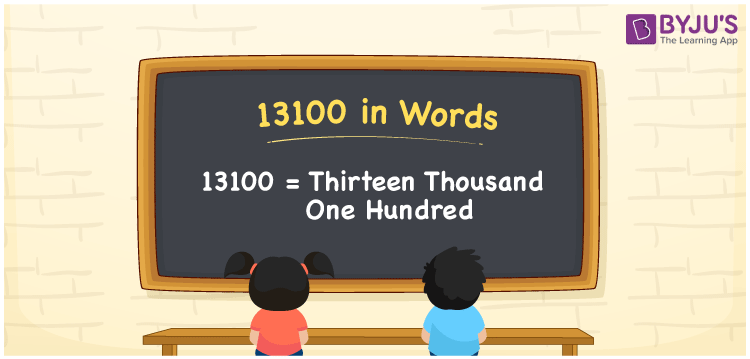# 13100 in Words

The name of the number 13100 in words is Thirteen Thousand One Hundred. For example, if you purchased a golden bracelet worth Rs.13100, then you can say, “I purchased a golden bracelet worth Rupees Thirteen Thousand One Hundred”. We know that 13100 is a cardinal number since it denotes a certain amount. In this article, let us learn how to write the number name for the number 13100 with the help of a place value chart.

 13100 in Words Thirteen Thousand One Hundred Thirteen Thousand One Hundred in numerical form 13100

## 13100 in English Words

Generally, we write the numbers in words using the English alphabet since English is the most common language in the education field. Hence, we can write the number 13100 in English words as Thirteen Thousand One Hundred.## How to Write 13100 in Words?

It is essential to identify the place value of the number before converting it into words. The following table represents the place value chart for the number 13100.

 Ten-Thousands Thousands Hundreds Tens Ones 1 3 1 0 0

Hence, we can write the expanded form as:

1 x Ten Thousand + 3 x Thousand + 1 x Hundred + 0 x Ten + 0 x One

= 1 x 10000 + 3 x 1000 + 1 x 100 + 0 x 10 + 0 x 1

= 10000 + 3000 + 100 + 0 + 0

= 10000 + 3000 + 100

= 13100

= Thirteen Thousand One Hundred

Therefore, 13100 in words is written as Thirteen Thousand One Hundred

Interesting way of writing 13100 in words

1 = One

13 = Thirteen

131 = One Hundred and Thirty-One

1310 = One Thousand Three Hundred Ten

13100 = Thirteen Thousand One Hundred

Thus, the word form of the number 13100 is Thirteen Thousand One Hundred

13100 is a natural number that is the successor of 13099 and the predecessor of 13101

• 13100 in words – Thirteen Thousand One Hundred
• Is 13100 an odd number? – No
• Is 13100 an even number? – Yes
• Is 13100 a perfect square number? – No
• Is 13100 a perfect cube number? – No
• Is 13100 a prime number? – No
• Is 13100 a composite number? – Yes

## Frequently Asked Questions on 13100 in Words

Q1

### Write 13100 in words.

13100 in words is written as Thirteen Thousand One Hundred.
Q2

### Simplify 14100 – 1000, and express in words.

Simplifying 14100 – 1000, we get 13100. Hence, 13100 in words is Thirteen Thousand One Hundred.
Q3

### 13100 is an even number. True or False.

True, the number 13100 is an even number.